Home > ACC6 > Chapter cc17 > Lesson cc17.3.2 > Problem7-96

7-96.

On your paper, copy the chart below. Then complete two trials by reading the algebraic expressions. Write in the steps. Homework Help ✎

 Steps Trial 1 Trial 2 Algebraic Expression 1. $x$ 2. $6x$ 3. $6x + 24$ 4. $6x + 18$ 5. $x + 3$ 6. $3$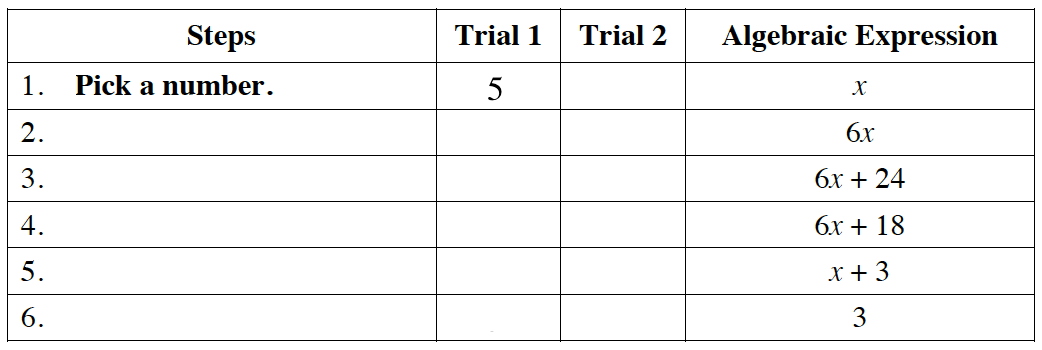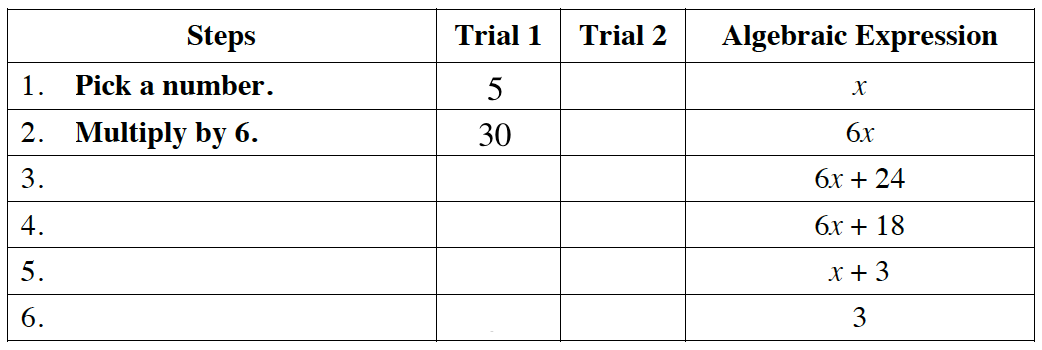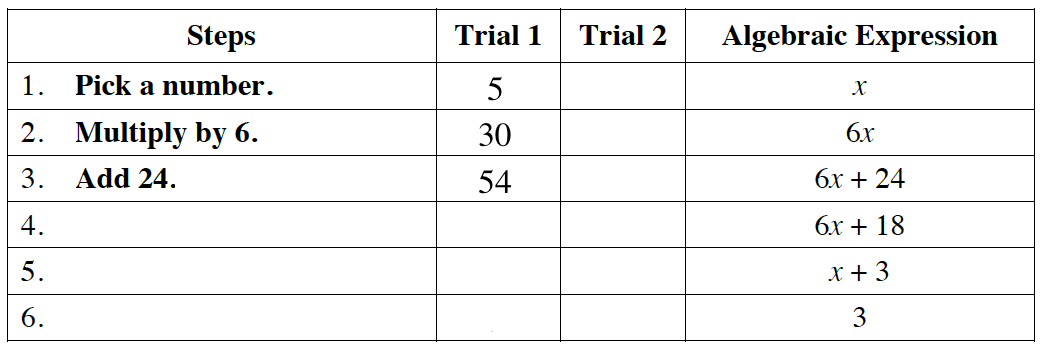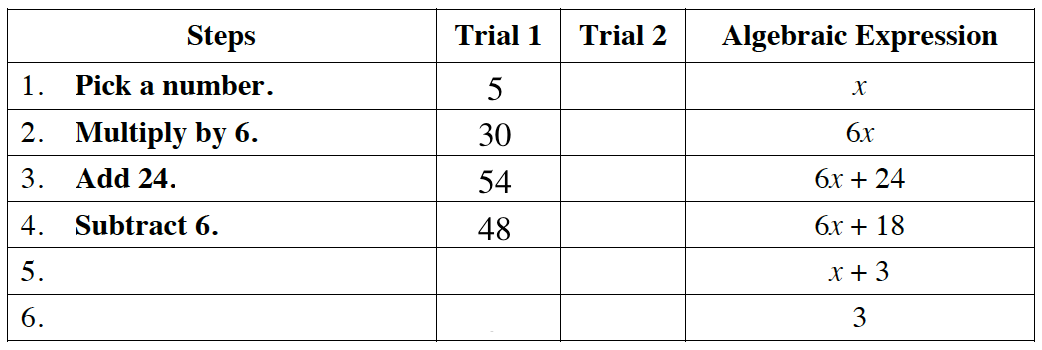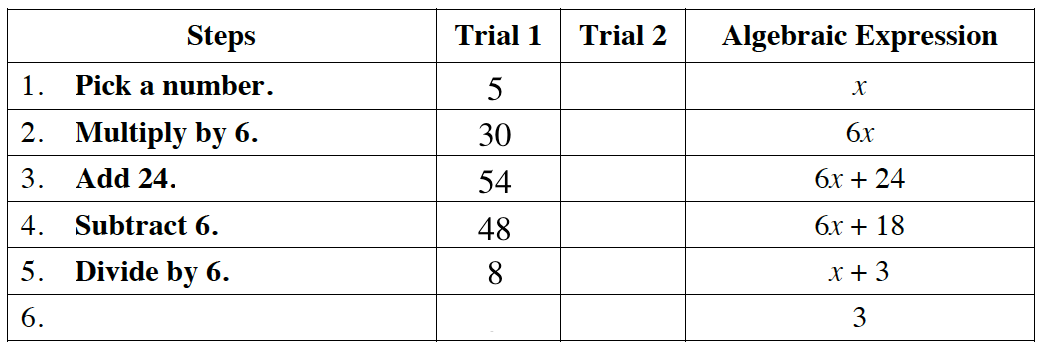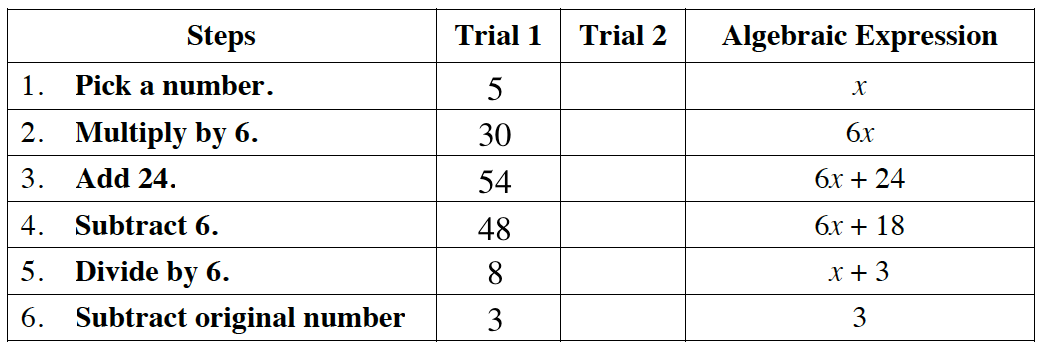Now, go back and pick a different number for the 2nd trial.
Follow the same steps!